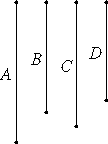# Proposition 27

To find medial straight lines commensurable in square only which contain a rational rectangle.

Set out two rational straight lines A and B commensurable in square only. Take a mean proportional C between A and B. Let it be contrived that A is to B as C is to D.Then, since A and B are rational and commensurable in square only, therefore the rectangle A by B, that is, the square on C, is medial. Therefore C is medial.

X.11

And since A is to B as C is to D, and A and B are commensurable in square only, therefore C and D are also commensurable in square only.

X.23.Note

And C is medial, therefore D is also medial.

Therefore C and D are medial and commensurable in square only.

I say that they also contain a rational rectangle.

V.16

Since A is to B as C is to D, therefore, alternately, A is to C as B is to D.

But A is to C as C is to B, therefore C is to B as B is to D. Therefore the rectangle C by D equals the square on B. But the square on B is rational, therefore the rectangle C by D is also rational.

Therefore medial straight lines commensurable in square only have been found which contain a rational rectangle.

Q.E.D.

## Guide

If we set A to have length 1, and B length √b, an irrational number where b is rational, then C has length b1/4 and D has length b3/4. Then C and D are medial and commensurable in square only, and the b1/4 by b3/4 rectangle they contain has area b, a rational number.

This proposition is not used in the rest of the Elements.